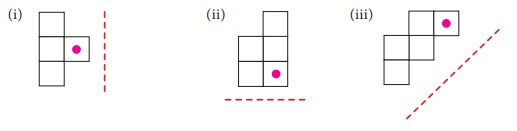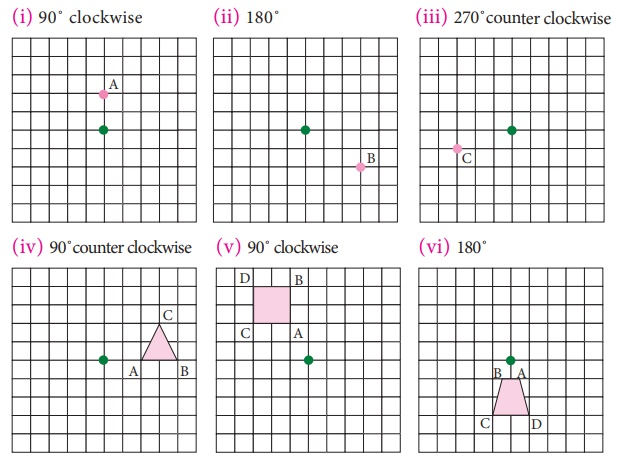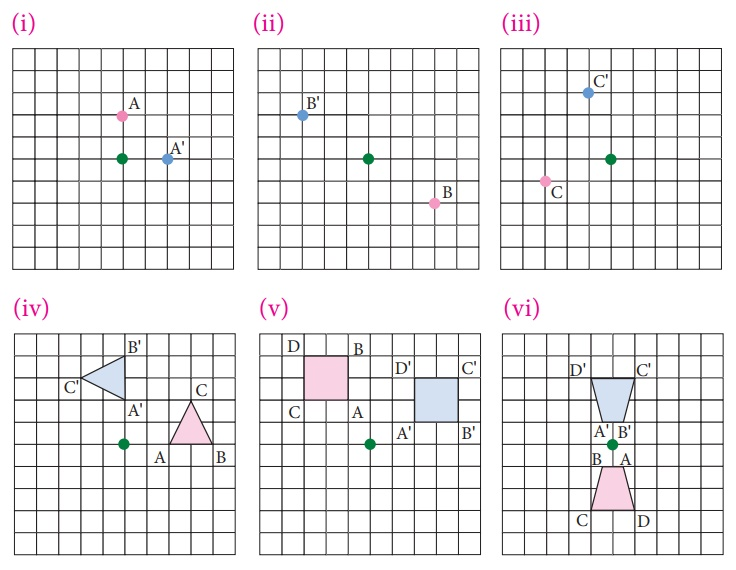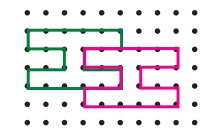Home | | Maths 7th Std | Exercise 4.1 (Symmetry through transformations)

# Exercise 4.1 (Symmetry through transformations)

7th Maths : Term 3 Unit 4 : Geometry : Symmetry through transformations : Text Book Back Exercises Questions with Answers, Solution

Exercise 4.1

1. Find the new position of each point using the translation given.

(i) 2→,4↓

(ii) 6 ↑

(iii) 3 ←, 5 ↓

(iv) 4 →, 3 ↑Solution:2. How is the pre-image translated to the image?Solution:

(i) 3 → , 4 ↑ (ii) 3 ←, 3 ↑

(iii) 4 ←, 4 ↓ (iv) 2 → , 2↓

3. Find the image of the given triangle with given translation.

(i) 4 ↑

(ii) 6 → 3 ↓

(iii) 5 ← 4 ↓

(iv) 4→3↑Solution:4. Reflect the shape with given line of reflection.Solution:5. Reflect the shape in each of the following pictures with given line of reflection.Solution:6. Rotate the preimages in each case as directed about the green point.

(i) 90˚ clockwise (ii) 180° (iii) 270˚counter clockwise (iv) 90˚counter clockwise (v) 90˚ clockwise (vi) 180˚Solution:Identify the transformation

7.Solution:  Reflection

8.Solution:  Rotation

9.Solution:  Translation

10. A pool of fish translates from point F to point D.a. Describe the translation of the pool of fish.

b. Can the fishing boat make the same translation? Explain.

c. Describe a translation the fishing boat could make to get to point D.

Solution:

(a) Translation of pool of fish is 7→, 2 ↓

(b) No, the fishing boat will be landed on the island if translated.

(c) To get point D, the translation will be 5 →, 3↓

11. Name the transformation that will map footprint A onto the indicated footprint.(i) Footprint B

(ii) Footprint C

(iii) Footprint D

(iv) Footprint E

Solution:

(i) It is translation

12. In given diagram, the blue figure is an image of the pink figure.(i) Choose an angle or a vertex from the preimage and name its image.

(ii) List all pairs of corresponding sides.

Solution:

(i) Image of L is L', Image of M is M',

Image of N is N', Image of O is O'

Image of vertex L is L', Image of vertex M is M'

Image of vertex N is N', Image of vertex O is O'

(ii) Corresponding sides are LM and L'M', MN and M'N', NO and N'O' and OL and O'L'

13. In the diagram at the right, the green figure is a translation image of the pink figure. Write a coordinate rule that describes the translation.Solution:  The rule bind here in 3→, ↑↓

Objective type questions.

14. A _____ is a turn about a point.

(i) Translation

(ii) Rotation

(iii) Reflection

(iv) Glide Reflection

15. A _____ is a flip over a line.

(i) Translation

(ii) Rotation

(iii) Reflection

(iv) Glide Reflection

16. A _____ is a slide; move without turning or flipping the shape.

(i) Translation

(ii) Rotation

(iii) Reflection

(iv) Glide Reflection

17. The transformation used in the picture is(i) Translation

(ii)  Rotation

(iii) Reflection

(iv) Glide Reflection

18. The transformation used in the picture is(i) Translation

(ii)  Rotation

(iii) Reflection

(iv) Glide Reflection

19. You must rotate the puzzle piece 270° clockwise about the point P to fit it into a puzzle. Which piece fits in the puzzle as shown?[Answer: (iii)Exercise 4.1

1.2. (i) 3→,4↑ (ii) 3←, 3↑ (iii) 4←, 4↓ (iv) 2→,2↓

3.4.5.6.7. Reflection

8. Rotation

9. Translation

10. a. 7→,2↓

b. No. It will be landed on the island.

c. 5→,3↓

11. (i) Translation (ii) Reflection about horizontal line (iii) Reflection about vertical line (iv) Rotation about the heel

12. (i) Image of L is L’, Image of M is M’,

Image of N is N’, Image of O is O’

Image of vertex L is L’, Image of vertex M is M’

Image of vertex N is ∠N; Image of vertex O is O’

(ii) Corresponding sides are LM and L’M’, MN and M’N’, NO and N’O’ and OL and O’L’

13. 3→ 1↓

Objective type questions.

14. (ii) Rotation

15. (iii) Reflection

16. (i) Translation

17. (ii) Rotation

18.(i) Translation

19.(iii)Tags : Geometry | Term 3 Chapter 4 | 7th Maths , 7th Maths : Term 3 Unit 4 : Geometry
Study Material, Lecturing Notes, Assignment, Reference, Wiki description explanation, brief detail
7th Maths : Term 3 Unit 4 : Geometry : Exercise 4.1 (Symmetry through transformations) | Geometry | Term 3 Chapter 4 | 7th Maths